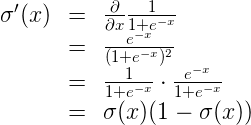# Lesson 2: Introduction to Neural Networks_II

#### – Logistic Regression:

• Pick a random model
• Calculate the error
• Minimize the error, and obtain a better model

#### – Calculating the Error Function#### Day 25 of #60daysofudacitysigmoid function :